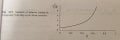# Confused over an easy plot about inductor current

Thread Starter

#### N9vem

Joined Jul 12, 2017
1Need some insight about a plot found in the Fundamentals of power electronics second edition book.
I don't really understand why it is written like this. Left side $$\frac{I}{V_{g}/R}$$ can be rewritten to $$\frac{1}{D'^{2}}$$ so it is basically "just" duty cycle.

The book states that the equation plotted is $$I=\frac{V_{g}}{D'^{2} R}$$

I know I'm missing something obvious but my mind just jammed in this, so if you can help, please enlighten me.

Its on page 25 of fundamentals of power electronics second edition (Robert W Erickson, D. Maksimovic) 2.3 boost converter example chapter
Thank you!

#### puppydog846

Joined Apr 5, 2020
11
View attachment 203581
Need some insight about a plot found in the Fundamentals of power electronics second edition book.
I don't really understand why it is written like this. Left side $$\frac{I}{V_{g}/R}$$ can be rewritten to $$\frac{1}{D'^{2}}$$ so it is basically "just" duty cycle.

The book states that the equation plotted is $$I=\frac{V_{g}}{D'^{2} R}$$

I know I'm missing something obvious but my mind just jammed in this, so if you can help, please enlighten me.

Its on page 25 of fundamentals of power electronics second edition (Robert W Erickson, D. Maksimovic) 2.3 boost converter example chapter
Thank you!

#### puppydog846

Joined Apr 5, 2020
11
i believe you mixed d’ = (1-D) that matches your curve i it a boost converter